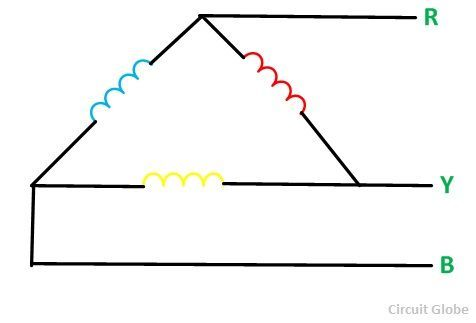# Difference Between Star and Delta Connection

Difference between star and delta connection are explained considering various factors like the basic definition of the connections, the existence of a neutral point, the connection of the terminals, the relation between line current and phase current and also between line voltage and phase voltage, speed, its insulation level, number of turns, type of system and network usage, etc.

The difference between the Star and Delta Connection are given below in the tabulated form.

BASISSTAR CONNECTIONDELTA CONNECTION

Basic DefinitionThe terminals of the three branches are connected to a common point. The network formed is known as Star Connection.The three branches of the network are connected in such a way that it forms a closed loop known as Delta Connection.
Connection of terminalsThe starting and the finishing point that is the similar ends of the three coils are connected together.The end of each coil is connected to the starting point of the other coil that means the opposite terminals of the coils are connected together.
Neutral pointNeutral or the star point exists in the star connection.Neutral point does not exist in the delta connection.
Relation between line and phase currentLine current is equal to the Phase current.Line current is equal to root three times of the Phase Current.
Relation between line and phase voltageLine voltage is equal to root three times of the Phase VoltageLine voltage is equal to the Phase voltage.
Speed The Speed of the star connected motors is slow as they receive 1/√3 of the voltage.The Speed of the delta connected motors is high because each phase gets the total of the line voltage.
Phase voltagePhase voltage is low as 1/√3 times of the line voltage.Phase voltage is equal to the line voltage.
Number of turnsRequires less number of turnsRequires large number of turns.
Insulation levelInsulation required is low.High insulation is required.
Network TypeMainly used in the Power Transmission networks.Used in the Power Distribution networks.
Type of systemBoth three phase four wire and three phase three wire system can be derived in star connection.Three phase four wire system can be derived from the Delta connection.

In this article, the difference between Star and Delta Connection is explained. In a three-phase circuit, there are two types of connections. One is known as Star Connection, and the other one is Delta Connection. A star connection has a common or a star point to which all the three terminals are connected forming a star shape as shown below:In delta connection, all the three terminals are connected together forming a closed loop. In this, there is no common or neutral point, and it is used for power transmission for short distances. The connection diagram is shown below:## Difference Between Star and Delta Connection

1. The terminals of the three branches are connected to a common point. The network formed is known as Star Connection. The three branches of the network are connected in such a way that it forms a closed loop known as Delta Connection.
2. In a star connection, the starting and the finishing point ends of the three coils are connected together to a common point known as the neutral point. But in Delta connection, there is no neutral point. The end of each coil is connected to the starting point of the other coil that means the opposite terminals of the coils are connected together.
3. In star connection, the line current is equal to the phase current, whereas in delta connection the line current is equal to root three times of the phase current.
4. In Star connection, the line voltage is equal to root three times of the phase voltage, whereas in delta connection line voltage is equal to the phase voltage.
5. The speed of the star-connected motors is slow as they receive 1/√3 of the voltage but the speed of the delta connected motors is high because each phase gets the total of the line voltage.
6. In star connection, phase voltage is low as 1/√3 times the line voltage, whereas in delta connection phase voltage is equal to the line voltage.
7. Star connections are mainly required for the Power Transmission Network for longer distances, whereas in delta connection mainly in Distribution networks and is used for shorter distances.
8. In star connection, each winding receives 230 volts and in delta connection, each winding receives 415 volts.
9. Both 3 phase 4 wire and 3 phase 3 wire systems can be derived in the star connection, whereas in Delta Connection only 3 phase 4 wire systems can be derived.
10. The amount of insulation required in star connection is low and in delta connection high insulation level is required.

These points differentiate between the star and delta connection.

### 17 thoughts on “Difference Between Star and Delta Connection”

1. MARK DICKENS OIMEKE

what about transformers are they connected in star form or delta form? and the 3 phase phoso mill is it delta connection or star connection?

1. The three-phase transformer is connected in fours ways.
1. Delta primary – Delta Secondary
2. Star Primary – Star Secondary
3. Delta Primary – Star Secondary
4. Star Primary – Delta Secondary
The choice of connections depends on the various factor.

2. help please, why are the secondary windings of a 3-phase- transformer, joined to high voltage distribution lines, joined in delta. economical factors must be taken into consideration.